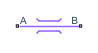# Flow Resistance (MA)

General resistance in a moist air branch

•Libraries:
Simscape / Foundation Library / Moist Air / Elements

## Description

The Flow Resistance (MA) block models a general pressure drop in a moist air network branch. The drop in pressure is proportional to the square of the mixture mass flow rate and inversely proportional to the mixture density. The constant of proportionality is determined from a nominal operating condition specified in the block dialog box. Set the Nominal mixture density parameter to zero to omit the density dependence.

Use this block when empirical data on the pressure losses and flow rates through a component is available, but detailed geometry information is unavailable.

The block equations use these symbols.

 $\stackrel{˙}{m}$ Mass flow rate Φ Energy flow rate p Pressure ρ Density R Specific gas constant S Cross-sectional area K Proportionality constant h Specific enthalpy T Temperature

Subscripts `a`, `w`, and `g` indicate the properties of dry air, water vapor, and trace gas, respectively. Subscripts `A` and `B` indicate the appropriate port.

Mass balance:

`$\begin{array}{l}{\stackrel{˙}{m}}_{A}+{\stackrel{˙}{m}}_{B}=0\\ {\stackrel{˙}{m}}_{wA}+{\stackrel{˙}{m}}_{wB}=0\\ {\stackrel{˙}{m}}_{gA}+{\stackrel{˙}{m}}_{gB}=0\end{array}$`

Energy balance:

`${\Phi }_{A}+{\Phi }_{B}=0$`

If the Nominal mixture density parameter value, ρnom, is greater than zero, then the block computes the pressure drop as

`${p}_{A}-{p}_{B}={K}_{1}{\stackrel{˙}{m}}_{A}\sqrt{{\stackrel{˙}{m}}_{A}^{2}+{\left({f}_{lam}{\stackrel{˙}{m}}_{nom}\right)}^{2}}\frac{R{T}_{in}}{{p}_{in}}$`

where:

• flam is the fraction of nominal mixture mass flow rate for laminar flow transition.

• pin is the inlet pressure (pA or pB, depending on flow direction).

• Tin is the inlet temperature (TA or TB, depending on flow direction).

The proportionality constant is computed from the nominal flow conditions as

`${K}_{1}=\frac{\Delta {p}_{nom}{\rho }_{nom}}{{\stackrel{˙}{m}}_{nom}^{2}}$`

If the Nominal mixture density parameter is set to zero, then the block computes the pressure drop as

`${p}_{A}-{p}_{B}={K}_{2}{\stackrel{˙}{m}}_{A}\sqrt{{\stackrel{˙}{m}}_{A}^{2}+{\left({f}_{lam}{\stackrel{˙}{m}}_{nom}\right)}^{2}}$`

where the proportionality constant is computed from the nominal flow conditions as

`${K}_{2}=\frac{\Delta {p}_{nom}}{{\stackrel{˙}{m}}_{nom}^{2}}$`

The flow resistance is assumed adiabatic, so the mixture specific total enthalpies are equal

`${h}_{A}+\frac{1}{2}\left(\frac{{\stackrel{˙}{m}}_{A}R{T}_{A}}{S{p}_{A}}\right)={h}_{B}+\frac{1}{2}\left(\frac{{\stackrel{˙}{m}}_{B}R{T}_{B}}{S{p}_{B}}\right)$`

### Assumptions and Limitations

• The resistance is adiabatic. It does not exchange heat with the environment.

• The pressure drop is assumed to be proportional to the square of the mixture mass flow rate and inversely proportional to the mixture density.

## Ports

### Conserving

expand all

Moist air conserving port associated with the inlet or outlet of the flow resistance. This block has no intrinsic directionality.

Moist air conserving port associated with the inlet or outlet of the flow resistance. This block has no intrinsic directionality.

## Parameters

expand all

Pressure drop from inlet to outlet at a known operating condition. The block uses the nominal parameters to calculate the constant of proportionality between the pressure drop and the mass flow rate.

Mass flow rate of the air mixture through the block at a known operating condition. The block uses the nominal parameters to calculate the constant of proportionality between the pressure drop and the mass flow rate.

Mass density of the moist air mixture inside the flow resistance at a known operating condition. The block uses the nominal parameters to calculate the constant of proportionality between the pressure drop and the mass flow rate. Set this parameter to zero to ignore the dependence of the pressure drop on the air mixture density.

Flow area at the ports of the flow resistance. The ports are assumed to be identical in size.

Ratio of the threshold mass flow rate to the nominal mass flow rate of the air mixture. The block uses this parameter to set the threshold for the linearization of the pressure drop.

## Version History

Introduced in R2018a